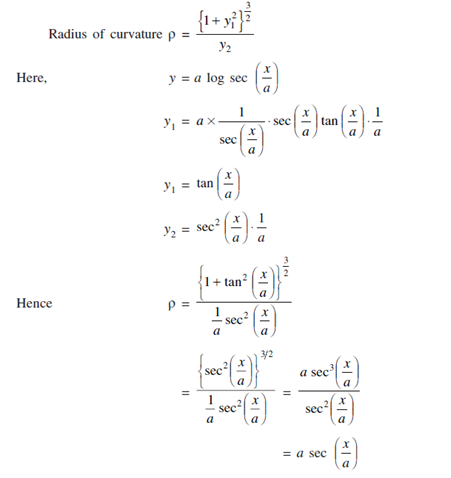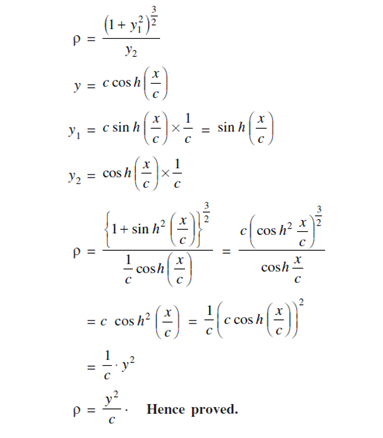1. Find the radius of curvature at any point on the curve y = a log sec ( x/a ).

Solutions:Radius of Curvature = a sec (x/a)

2. For the curve y = c cos h (x/c), show that ρ = y2/c

Solutions: